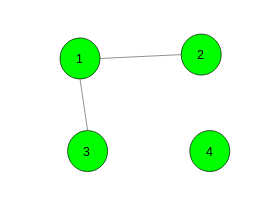# Number of ways to select a node from each connected component

Given a graph with N nodes and M edges. The task is to find the number of ways to select a node from each connected component of the given graph.

Examples:

Input:Output: 3
(1, 4), (2, 4), (3, 4) are possible ways.

Input:Output: 6
(1, 4, 5), (2, 4, 5), (3, 4, 5), (1, 4, 6), (2, 4, 6), (3, 4, 6) are possible ways.

## Recommended: Please try your approach on {IDE} first, before moving on to the solution.

Approach: A product of the number of nodes in each connected component is the required answer. Run a simple dfs to find the number of nodes in each connected component.

Below is the implementation of the above approach:

## C++

 `// C++ implementation of the approach ` `#include ` `using` `namespace` `std; ` `#define N 100005 ` ` `  `int` `n, m, temp; ` `vector<``int``> gr[N]; ` `int` `vis[N]; ` ` `  `// Function to add edges in the graph ` `void` `Add_edges(``int` `x, ``int` `y) ` `{ ` `    ``gr[x].push_back(y); ` `    ``gr[y].push_back(x); ` `} ` ` `  `// Function for DFS ` `void` `dfs(``int` `ch) ` `{ ` `    ``// Mark node as visited ` `    ``vis[ch] = 1; ` ` `  `    ``// Count number of nodes in a component ` `    ``temp++; ` `    ``for` `(``auto` `i : gr[ch]) ` `        ``if` `(!vis[i]) ` `            ``dfs(i); ` `} ` ` `  `// Function to return the required number of ways ` `int` `NumberOfWays() ` `{ ` ` `  `    ``// To store the required answer ` `    ``int` `ans = 1; ` ` `  `    ``memset``(vis, 0, ``sizeof` `vis); ` `    ``for` `(``int` `i = 1; i <= n; i++) { ` ` `  `        ``// If current node hasn't been visited yet ` `        ``if` `(!vis[i]) { ` `            ``temp = 0; ` `            ``dfs(i); ` ` `  `            ``// Multiply it with the answer ` `            ``ans *= temp; ` `        ``} ` `    ``} ` ` `  `    ``return` `ans; ` `} ` ` `  `// Driver code ` `int` `main() ` `{ ` `    ``n = 4, m = 2; ` ` `  `    ``// Add edges ` `    ``Add_edges(1, 2); ` `    ``Add_edges(1, 3); ` ` `  `    ``cout << NumberOfWays(); ` ` `  `    ``return` `0; ` `} `

## Python3

 `# Python3 implementation of the approach ` ` `  `# Function to add edges in the graph ` `def` `Add_edges(x, y): ` ` `  `    ``gr[x].append(y) ` `    ``gr[y].append(x) ` ` `  `# Function for DFS ` `def` `dfs(ch): ` ` `  `    ``# Mark node as visited ` `    ``vis[ch] ``=` `1` `    ``global` `temp ` `     `  `    ``# Count number of nodes  ` `    ``# in a component ` `    ``temp ``+``=` `1` `    ``for` `i ``in` `gr[ch]: ` `        ``if` `not` `vis[i]: ` `            ``dfs(i) ` ` `  `# Function to return the required  ` `# number of ways ` `def` `NumberOfWays(): ` ` `  `    ``# To store the required answer ` `    ``ans ``=` `1` `    ``global` `temp ` `     `  `    ``for` `i ``in` `range``(``1``, n ``+` `1``):  ` ` `  `        ``# If current node hasn't been  ` `        ``# visited yet ` `        ``if` `not` `vis[i]: ` `            ``temp ``=` `0` `            ``dfs(i) ` ` `  `            ``# Multiply it with the answer ` `            ``ans ``*``=` `temp ` `         `  `    ``return` `ans ` ` `  `# Driver code ` `if` `__name__ ``=``=` `"__main__"``: ` ` `  `    ``n, m, temp ``=` `4``, ``2``, ``0` `    ``N ``=` `100005` ` `  `    ``gr ``=` `[[] ``for` `i ``in` `range``(N)] ` `    ``vis ``=` `[``None``] ``*` `N ` ` `  `    ``# Add edges ` `    ``Add_edges(``1``, ``2``) ` `    ``Add_edges(``1``, ``3``) ` ` `  `    ``print``(NumberOfWays()) ` ` `  `# This code is contributed by Rituraj Jain `

Output:

```3
```

My Personal Notes arrow_drop_uppawanasipugmailcom

If you like GeeksforGeeks and would like to contribute, you can also write an article using contribute.geeksforgeeks.org or mail your article to contribute@geeksforgeeks.org. See your article appearing on the GeeksforGeeks main page and help other Geeks.

Please Improve this article if you find anything incorrect by clicking on the "Improve Article" button below.

Improved By : rituraj_jain